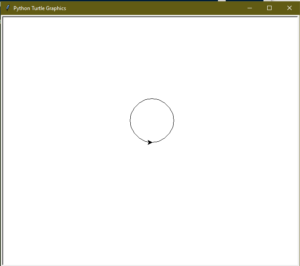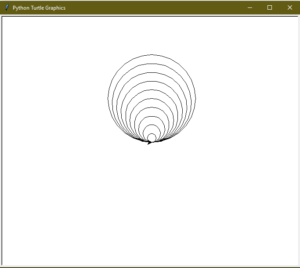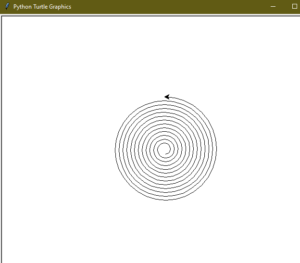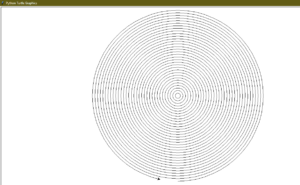# Draw Circle in Python using Turtle

Turtle is a Python feature like a drawing board, which lets us command a turtle to draw all over it! We can use functions like `turtle.forward(…)` and `turtle.right(…)` which can move the turtle around. Turtle is a beginner-friendly way to learn Python by running some basic commands and viewing the turtle do it graphically. It is like a drawing board that allows you to draw over it. The turtle module can be used in both object-oriented and procedure-oriented ways.

To draw, Python `turtle` provides many functions and methods i.e. forward, backward, etc. Some the commonly used methods are:

• forward(x): moves the pen in the forward direction by x unit.
• backward(x): moves the pen in the backward direction by x unit.
• right(x): rotate the pen in the clockwise direction by an angle x.
• left(x): rotate the pen in the anticlockwise direction by an angle x.
• penup(): stop drawing of the turtle pen.
• pendown(): start drawing of the turtle pen.

Now to draw a circle using turtle, we will use a predefined function in “turtle”.

circle(radius): This function draws a circle of the given radius by taking the “turtle” position as the center.

Example:

 `# Python program to demonstrate ` `# circle drawing ` ` `  ` `  `import` `turtle ` `   `  `# Initializing the turtle ` `t ``=` `turtle.Turtle() ` ` `  ` `  `r ``=` `50` `t.circle(r) `

Output :#### Tangent Circles

A tangent is a line that touches the circumference of a circle from outside at a point, provided that any extension of the line will not cause intersection with the circle. Now, think about a group of circles, that have a common tangent. The group of circles, having common tangent, are known as tangent circles.

Example:

 `# Python program to demonstrate ` `# tangent circle drawing ` ` `  ` `  `import` `turtle ` `   `  `t ``=` `turtle.Turtle() ` ` `  `# radius for smallest circle ` `r ``=` `10` ` `  `# number of circles ` `n ``=` `10` ` `  `# loop for printing tangent circles ` `for` `i ``in` `range``(``1``, n ``+` `1``, ``1``): ` `    ``t.circle(r ``*` `i) `

Output :#### Spiral Circle

Spiral is a shape similar to a circle, except that the radius of the spiral gradually increases after every completed round.

Exmaple:

 `# Python program to demonstrate ` `# spiral circle drawing ` ` `  ` `  `import` `turtle ` `   `  `t ``=` `turtle.Turtle() ` ` `  `# taking radius of initial radius ` `r ``=` `10` ` `  `# Loop for printing spiral circle ` `for` `i ``in` `range``(``100``): ` `    ``t.circle(r ``+` `i, ``45``) `

Output :#### Cocentric Circles

The term concentric is used for a group of things having common. Now Circles having the same center are termed as Concentric Circle.

 `# Python program to demonstrate ` `# concentric circle drawing ` ` `  ` `  `import` `turtle ` `   `  `     `  `t ``=` `turtle.Turtle() ` ` `  `# radius of the circle ` `r ``=` `10` ` `  `# Loop for printing concentric circles ` `for` `i ``in` `range``(``50``): ` `    ``t.circle(r ``*` `i) ` `    ``t.up() ` `    ``t.sety((r ``*` `i)``*``(``-``1``)) ` `    ``t.down() `

Output :My Personal Notes arrow_drop_upCheck out this Author's contributed articles.

If you like GeeksforGeeks and would like to contribute, you can also write an article using contribute.geeksforgeeks.org or mail your article to contribute@geeksforgeeks.org. See your article appearing on the GeeksforGeeks main page and help other Geeks.

Please Improve this article if you find anything incorrect by clicking on the "Improve Article" button below.

Article Tags :

3

Please write to us at contribute@geeksforgeeks.org to report any issue with the above content.